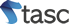# Archimedes Screw

Freeflow69 has worked in collaboration withto develop the Archimedes Screw turbine.

The Archimedes Screw is a revolutionary Hydroelectric Turbine design based on the Greek Archimedes Screw principles. It is a fish friendly, efficient device for converting the potential energy of flowing water into usable electricity. The turbine is made from reinforced plastic and is suitable for small to medium domestic or commercial sites producing up to 15kw. The Turbine is designed and manufactured here in the UK and is transported and installed simply and easily with minimal construction work.

Most other Archimedes Screw Turbines currently available in the market are constructed from metal, making them more expensive to manufacture, transport and install. These metal versions are more suitable for larger scale sites, for generating 50Kw or more.

## How do you know if you have a site suitable for generating electricity?

To establish if your site is suitable for generating electricity from aa Archimedes Screw you need to have flowing water and you need to know the three key components, which are WATER VELOCITY (in metres per second), AREA (in square metres) and HEAD (in metres).

### Water Velocity

The more flowing water you have, the more potential power you can generate. The Water Velocity can be estimated by recording how long (in seconds) a ‘floating object’ (such as a ball) takes to travel over a given distance (in metres). Divide the distance by the time taken and you will have the Water Velocity (in metres per second).

### Area

The Area is calculated using the simple equation of:
Depth (in metres) x Width (in metres) = Area (in square metres)

The water needs to drop, or fall, a distance of between 1 and 5 metres. Measure how far the water falls in metres.

### Generated Power

To calculate the power generated by the above figures use the following equation which will estimate the potential power:

Water Velocity(metres per second) x Area (in square metres) x Head (in metres) x 9.81 (Constant for Gravity) = Potential Energy (in kilowatts)

### Efficiency

Most screws work on an efficiency of up to 80% (other efficiencies can be obtained from our Poncelet Wheels) using this efficiency value (i.e. 0.8) would give you the expected output from the site.

Potential Energy (in kilowatts) x Efficiency = Output Power